# Test: Electronic Devices - 2

## 20 Questions MCQ Test GATE ECE (Electronics) 2023 Mock Test Series | Test: Electronic Devices - 2

Description
Attempt Test: Electronic Devices - 2 | 20 questions in 60 minutes | Mock test for Electronics and Communication Engineering (ECE) preparation | Free important questions MCQ to study GATE ECE (Electronics) 2023 Mock Test Series for Electronics and Communication Engineering (ECE) Exam | Download free PDF with solutions
QUESTION: 1

### For a P-channel Ge JFET, it is having doping concentration of Na = 1.77 × 1015 cm−3 and Nd = 3.53 × 1018 per cm3, If channel thickness is a = 1 µ met. (Given Relative permittivity of Ge = 16 at T = 300° K)  Q. What is value of internal pinch-off voltage?

Solution: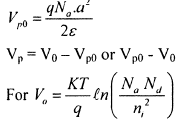QUESTION: 2

### For a P-channel Ge JFET, it is having doping concentration of Na = 1.77 × 1015 cm−3 and Nd = 3.53 × 1018 per cm3, If channel thickness is a = 1 µ met. (Given Relative permittivity of Ge = 16 at T = 300° K)  Q. What is value of pinch-off voltage?

Solution:QUESTION: 3

### If saturation currents of 2 diodes are 1 µA and 2 µA. If break down voltages of diode are same and are equal to 100 volt, what is value of current in D1.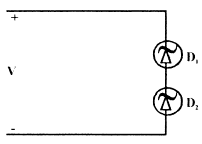Q. If V = 90 volt

Solution:
QUESTION: 4

If saturation currents of 2 diodes are 1 µA and 2 µA.
If break down voltages of diode are same and are equal to 100 volt, what is value of current in D1.Q.

If V = 110 volt

Solution:
QUESTION: 5

For Si transistor, if β ≥ 30 and ICBO = 10 nA  The minimum value of R, for transistor to remain in active region for vi = 12 volt, is __________ kΩ.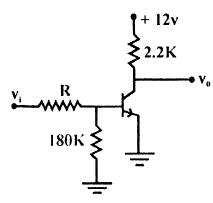Solution:
QUESTION: 6

A sample of silicon (uniformly doped n-type) at T = 300° K has the electron concentration varying linearly with distance as shown in the figure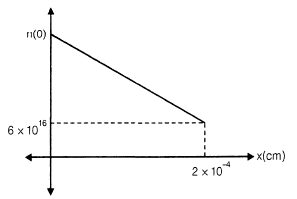The diffusion current is found to be −1120 A/cm2. If the diffusion constant Dn = 35 cm2/s, the electron concentration at x = 0 is

Solution: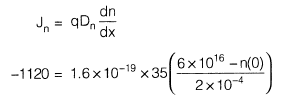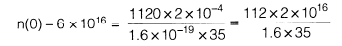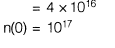QUESTION: 7

In a semiconductor sample,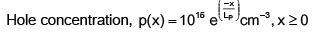where LP (hole diffusion length) = 4.8 × 10−4 cm

Hole diffusion coefficient DP = 20 cm2/s

The hole diffusion current density at x = 0 is

Solution: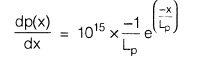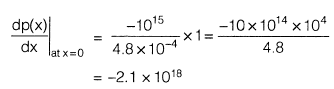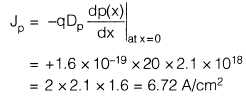QUESTION: 8

In the plot of log I vs V for a semiconductor Ge diode, the slope at room temperature is _________. Assume V >> VT and room temperature 27°C.

Solution: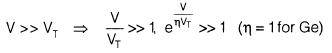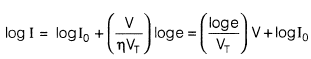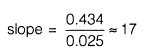QUESTION: 9

Consider a p-type semiconductor which is lightly doped i.e. condition of p >> n is not valid. p, n, ni are holes, electrons and intrinsic carrier concentration respectively. Then 2n + NA is ___________ if NA is immobile acceptor ions concentration

Solution:

According to charge neutrality,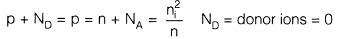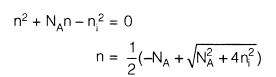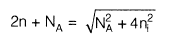QUESTION: 10

Consider the following network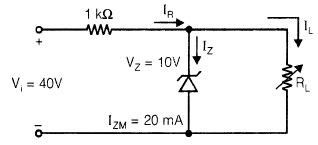VL is to be maintained at 10 V.
The correct representation of VL versus RL is

Solution: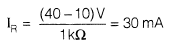RLmin to turn-on the zener diode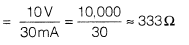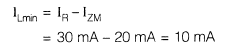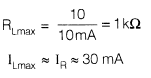QUESTION: 11

Consider the following sentences in respect of LEDs (Light emitting diodes) and semiconductor laser diode.
S1 :  Only direct band gap type semiconductors are suitable for fabrication of LEDs.
S2 : Both direct band gap type and indirect band gap type semiconductors are suitable for fabrication of semiconductor laser diode.
Choose the best alternative

Solution:

For the fabrication of semiconductor laser diode, direct band gap type semiconductor is required

QUESTION: 12

If individual JFET has IDSS = 5 mA and pinch-off voltage  VP0 = 3V ,  then current iD for VGS = − 0.9 V is __________ mA.

Solution: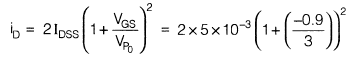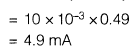QUESTION: 13

What are the states of three ideal diodes of circuit shown below: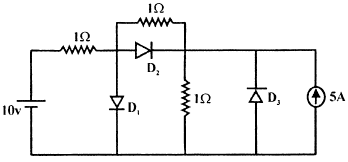Solution:
QUESTION: 14

For BJT, circuit shown assume that β of transistor is very large, if here Si transistor is used, then transistor will operate in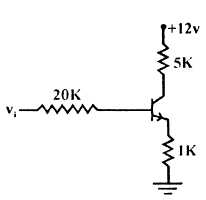Solution:

If β is large then transistor will be in S.R

QUESTION: 15

Consider the following circuit: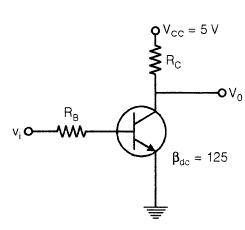Q.

If vi is as shown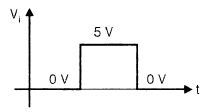then RB and RC for the circuit concerned can be _________ and _________ respectively, if ICsat = 6 mA. Assume the transistor inverter operation

Solution: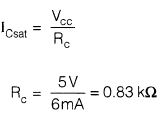Just at onset of saturation,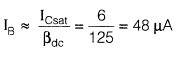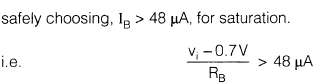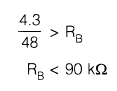QUESTION: 16

Consider the following circuit:Q.

The V0 shall be represented by

Solution:

Transistor is switch in inverter operation.

QUESTION: 17

A BJT having β = 125 is biased at a dc collector current of 1.23 mA. The values of gm, re and rπ at the bias point are: (Assume temperature of operation = 25°C).

Solution: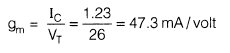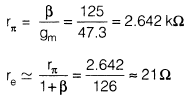QUESTION: 18

A properly biased JFET may be shown by one of the following figures.Choose the correct alternative: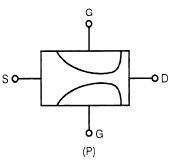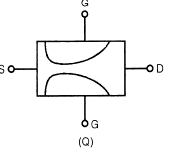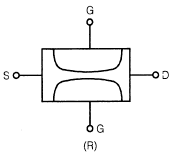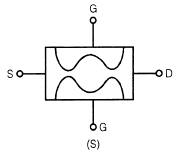Solution:

Because of the more reverse biased drain end, the depletion width is thinner in drain side.

QUESTION: 19

Shown below is the n-channel JFET in voltage divider bias arrangement.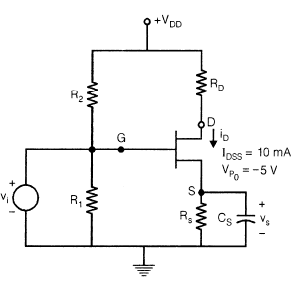VDD = 12 V
RS = RD = 1.8 kΩ

R1 = 100 kΩ

R2 = 400 kΩ

Q.

Gate to source voltage (VGS) is given by the quadratic equation

V2GS + PVGS + Q = 0
P and Q are respectively

Solution: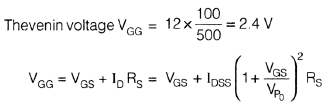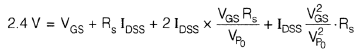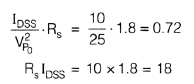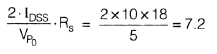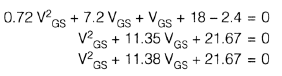QUESTION: 20

Shown below is the n-channel JFET in voltage divider bias arrangement.VDD = 12 V
RS = RD = 1.8 kΩ

R1 = 100 kΩ

R2 = 400 kΩ

Q.

The drain current iD is

Solution:

Solving VGS from the obtained quadratic equation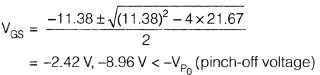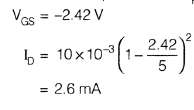Use Code STAYHOME200 and get INR 200 additional OFF Use Coupon Code